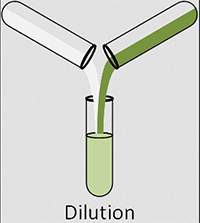# How To Calculate A Dilution### The simple formula of C1V1 = C2V2 is a lifesaver for those who are wanting to do dilutions.

The formula for calculating a dilution is (C1) (V1) = (C2) (V2) where...

• C1 is the concentration of the starting solution.
• V1 is the volume of the starting solution.
• C2 is the concentration of the final solution.
• V2 is the volume of the final solution.

### Example

We want to dilute a 5 molar (M) solution with water to make 1 liter (L) of a 1M solution. In this case, we know C1, the concentration of the starting solution, C2, the desired final concentration, and V2, the desired final volume. Using the formula we can find how much of the starting solution (V1) we need to make 1 liter of a 1M final solution.